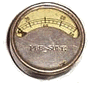# Perl Type Issues

Perl is a language with WeakTypeIssues.

In Perl there are is one single value type, the scalar. A scalar can represent a string, an integer or a double, and it is implementation uses these C types. But from Perl you can't tell which one it is using. Perl switches the format based on the usage context. For comparison of values, Perl has two sets of operators; string and numeric:

• eq, ne, gt, lt, etc
• ==, !=, >, <

DaveThomas posted a fit table to the fit-dev mailing list that demonstrates type ambiguity.

 Plusser a b plus 1 2 4 or should it be 4.0? x y xy concatenation vs addition

Here's how I resolve it in the AlternatePerlImplementation:

```    package Plusser;
use base 'Test::FIT::ColumnFixture';
sub a {
my (\$self, \$value) = @_;
\$self->{a} = \$value;
return;	       # no return value
}
sub b {
my (\$self, \$value) = @_;
# Alternate way to get value
\$self->{b} = \$self->cell->value;
return;	       # no return value
}
sub plus {
my (\$self, \$expected) = @_;
# Is value numeric?
if (\$self->{a} =~ /^-?[\d]+(\.\d*)?\$/) {
my \$actual = \$self->{a} + \$self->{b};
if (\$actual == \$expected) {
\$self->pass;
}
else {
\$self->fail("\$expected (expected) - \$actual (actual)");
}
}
else {
my \$actual = \$self->{a} . \$self->{b};
if (\$actual eq \$expected) {
\$self->pass;
}
else {
\$self->fail("\$expected (expected) - \$actual (actual)");
}
}
return;	       # no return value
}
1;
```
This demonstrates that I have a high level of control in my fixture, and am able to do the right thing no matter what my situation. But this is terribly verbose.

Fortunately I can make this much cleaner by upstreaming all the generic code into my base class. Here's the shorter revision:

```    package Plusser;
use base 'Test::FIT::ColumnFixture';
use Test::FIT qw(attribute);
attribute 'a';
attribute 'b';
sub plus {
my (\$self) = @_;
if (\$self->a =~ /^-?[\d]+(\.\d*)?\$/) {
\$self->eq_num(\$self->a + \$self->b);
}
else {
\$self->eq_str(\$self->a . \$self->b);
}
}
1;
```
This is about as clean as I can get it, and still retain the control I need.

Actually if 'a' and 'b' were 'x' and 'y', I could omit them because I keep a general purpose 'x', 'y', and 'z' in my base Fixture.pm class since they come up often :)

Last edited April 21, 2003